build_ts <- function( seed=123 )
{
set.seed( seed )

T = rep(1:365)
D = ifelse(T > 200, 1, 0)
P = ifelse(T <= 200, 0, rep(1:200))

err = rnorm(365, 150, 70)
Y = 5.4 + 0.5*T + 20*D + 1.2*P + err
Y <- rescale( Y, to = c(0, 300)) %>% round(2)
dat <- as.data.frame(cbind(Y, T, D, P))

return(dat)
}

dat.ts <- build_ts( seed=04172019 )  # seed set as date of assignment build

# build_rd  - regression discontinuity?
# build_iv  - instrumental variables

The dataset contains four variables:

Column Variable name Description
$$\text{Y}$$ $$\text{Wellbeing}$$ Wellbeing index (from 0 to 300)
$$\text{T}$$ $$\text{Time}$$ Time (from 1 to 365)
$$\text{D}$$ $$\text{Treatment}$$ Observation post (=1) and pre (=0) intervention
$$\text{P}$$ $$\text{Time Since Treatment}$$ Time passed since the intervention
Y T D P
1 95.43 1 0 0
2 87.76 2 0 0
3 30.46 3 0 0
4 15.78 4 0 0
5 114.14 5 0 0
6 4.34 6 0 0
7
198 172.05 198 0 0
199 111.36 199 0 0
200 211.07 200 0 0
201 130.53 201 1 1
202 145.76 202 1 2
203 64.19 203 1 3
204 121.07 204 1 4
15
360 265.31 360 1 160
361 260.68 361 1 161
362 267.22 362 1 162
363 232.51 363 1 163
364 296.84 364 1 164
365 275 365 1 165

Our model is based on the equation (??):

$\begin{equation} \text{Y} = \text{b}_0 + \text{b}_1*Time + \text{b}_2*Treatment + \text{b}_3*Time Since Treatment + \text{e} \tag{.} \end{equation}$

plot( dat.ts$T, dat.ts$Y, bty="n",
col=gray(0.5,0.5), cex=1.5, pch=19,
ylab="Well Being", xlab="Time (days)" )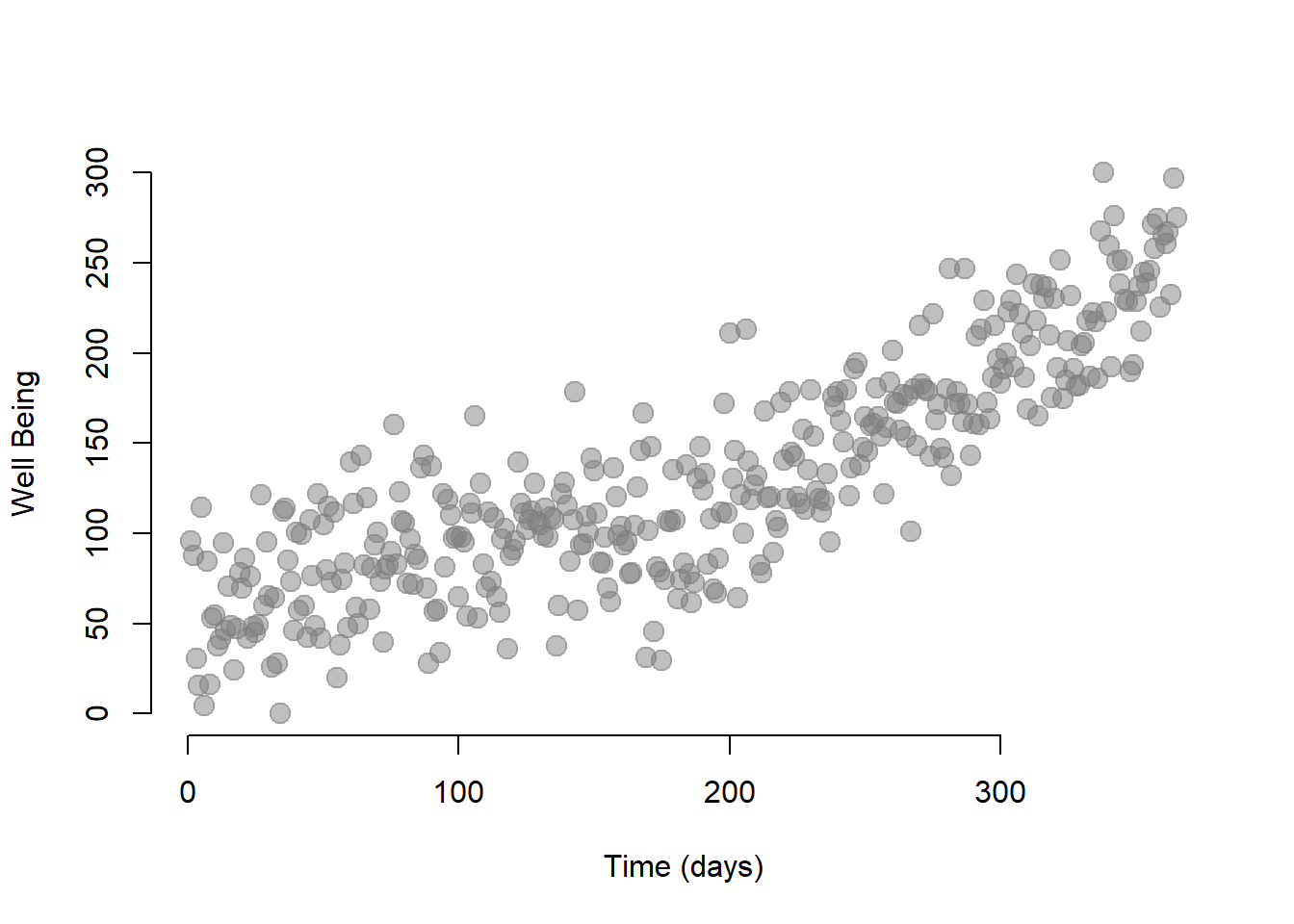We can run the model using the lm function in R.

regTS = lm ( Y ~ T + D + P, data=dat.ts )  # Our time series model

stargazer( regTS,
type = "html",
dep.var.labels = ("Wellbeing"),
column.labels = ("Model results"),
covariate.labels = c("Time", "Treatment", "Time Since Treatment"),
omit.stat = "all",
digits = 2 )
 Dependent variable: Wellbeing Model results Time 0.25*** (0.04) Treatment 1.03 (6.39) Time Since Treatment 0.57*** (0.06) Constant 62.75*** (4.31) Note: p<0.1; p<0.05; p<0.01

Let’s interpret our coefficients:

• The Time coefficient indicates the wellbeing trend before the intervention. It’s positive and significant, indicating that students’ wellbeing increases over time. For each day that passes, the wellbeing increases of 0.19 points on the index.

• The Treatment coefficient indicates the increase in the students’ wellbeing immediately after the intervention. We can see that the immediate effect is positive and significant indicating that attending the first class increased the students’ wellbeing of 13.09.

• The Time Since Treatment coefficient indicates that the trend has changed after the intervention. The sustained effect is positive and significant, indicating that for each day that passes after the intervention, the wellbeing of students increases of 0.54 points on the index.

## 0.1 Plotting the results

A useful exercise is to calculate outcomes at different points in time as we did in section ??. For instance, we can calculate the outcome right after the intervention, which occured at time = 200. Note that while $$\text{Time}$$ = 201, $$\text{Time Since Treatment}$$ is equal to 1 because it is the first day after the intervention.

$\begin{equation} \text{Y} = \text{b}_0 + \text{b}_1*201 + \text{b}_2*1 + \text{b}_3*1 + \text{e} \tag{.} \end{equation}$

We can also represent the point on a graph:

# We create a small dataset with the new values
data1 <- as.data.frame( cbind( T = 201, D = 1, P = 1 ))

# We use the function predict to (1) take the coefficients estimated in regTS and (2) calculate the outcome Y based on the values we set in the new datset
y1 <- predict( regTS, data1 )

# We plot our initial observations, the column Y in our dataset
plot( dat.ts$Y, col = "gray", xlim = c(1, 365), ylim = c(0, 400), xlab = "Time (days)", ylab = "Wellbeing index") # We add a point showing the level of wellbeing at time = 201) points(201, y1, col = "dodgerblue4", pch = 19, bg = "dodgerblue4", cex = 2) text(201, y1, labels = "t = 201", pos = 4, cex = 1) # Line marking the interruption abline( v=200, col="red", lty=2 )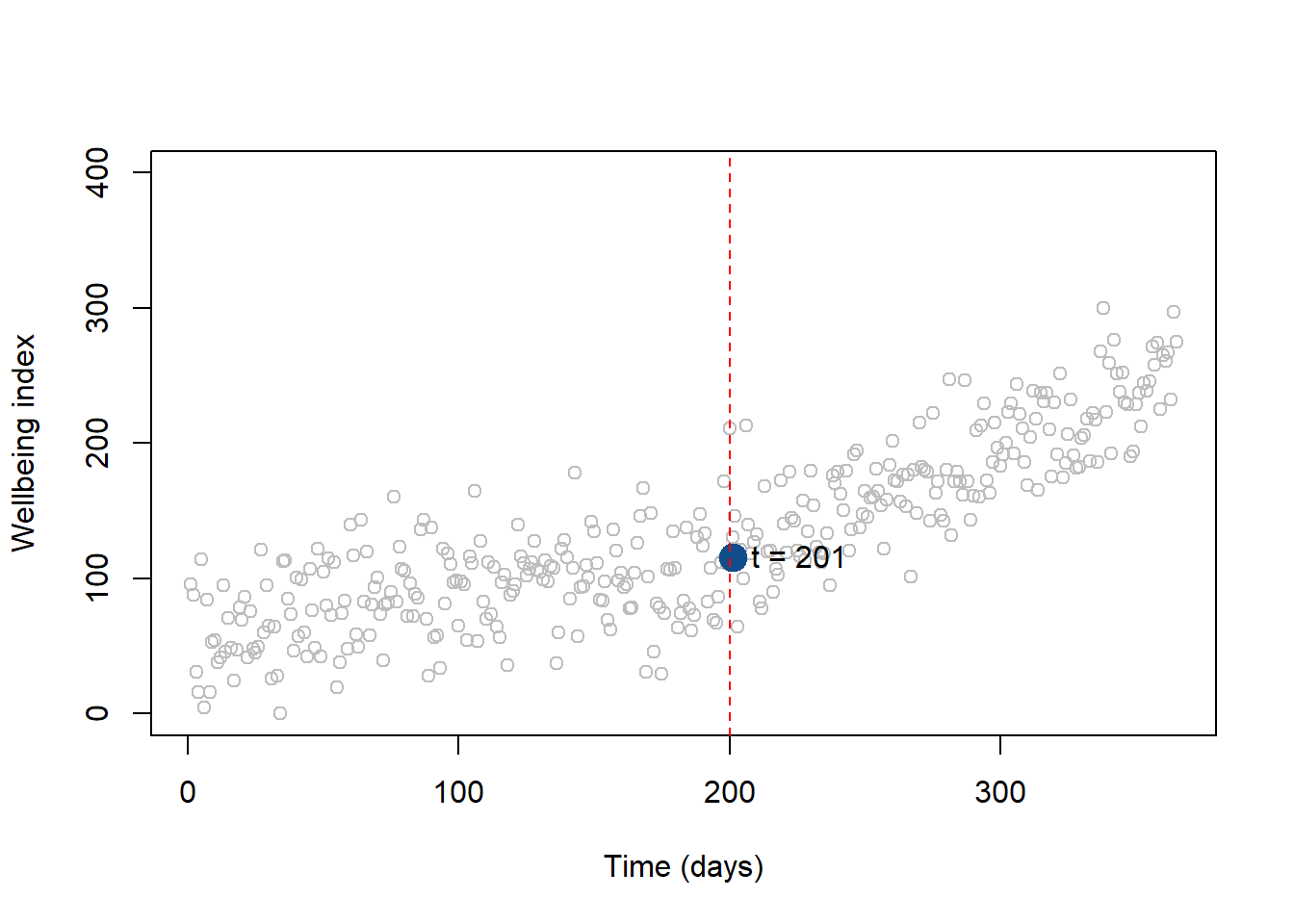Figure .: Wellbeing level at t = 201 # 1 Comparing Seeds dat1 <- build_ts( seed=123 ) dat2 <- build_ts( seed=456 ) jplot( dat1$Y, dat2$Y, xlab="Y", ylab="Y" )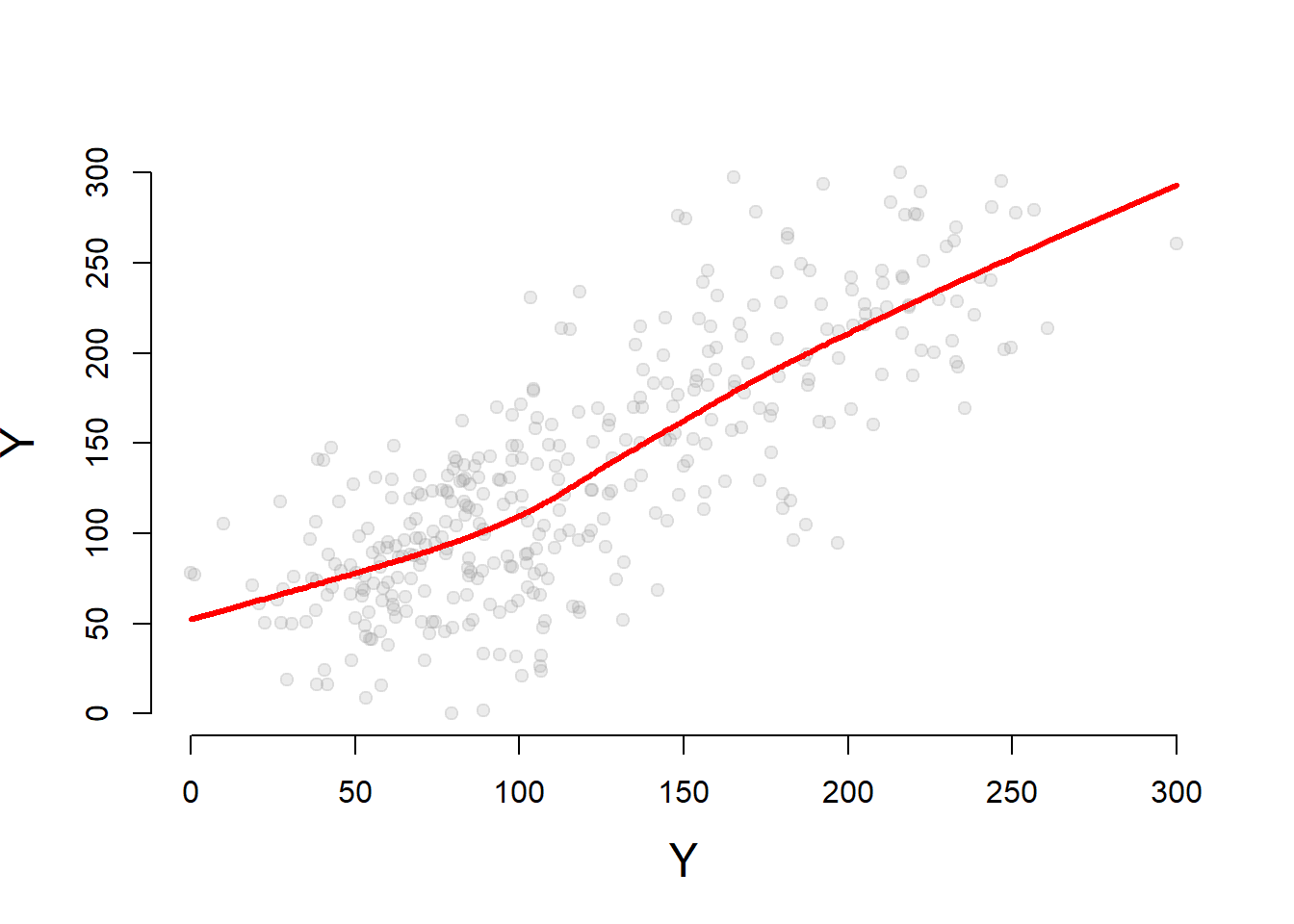jplot( dat1$T, dat2$T, main="T Not Stochastic" ) # not stochastic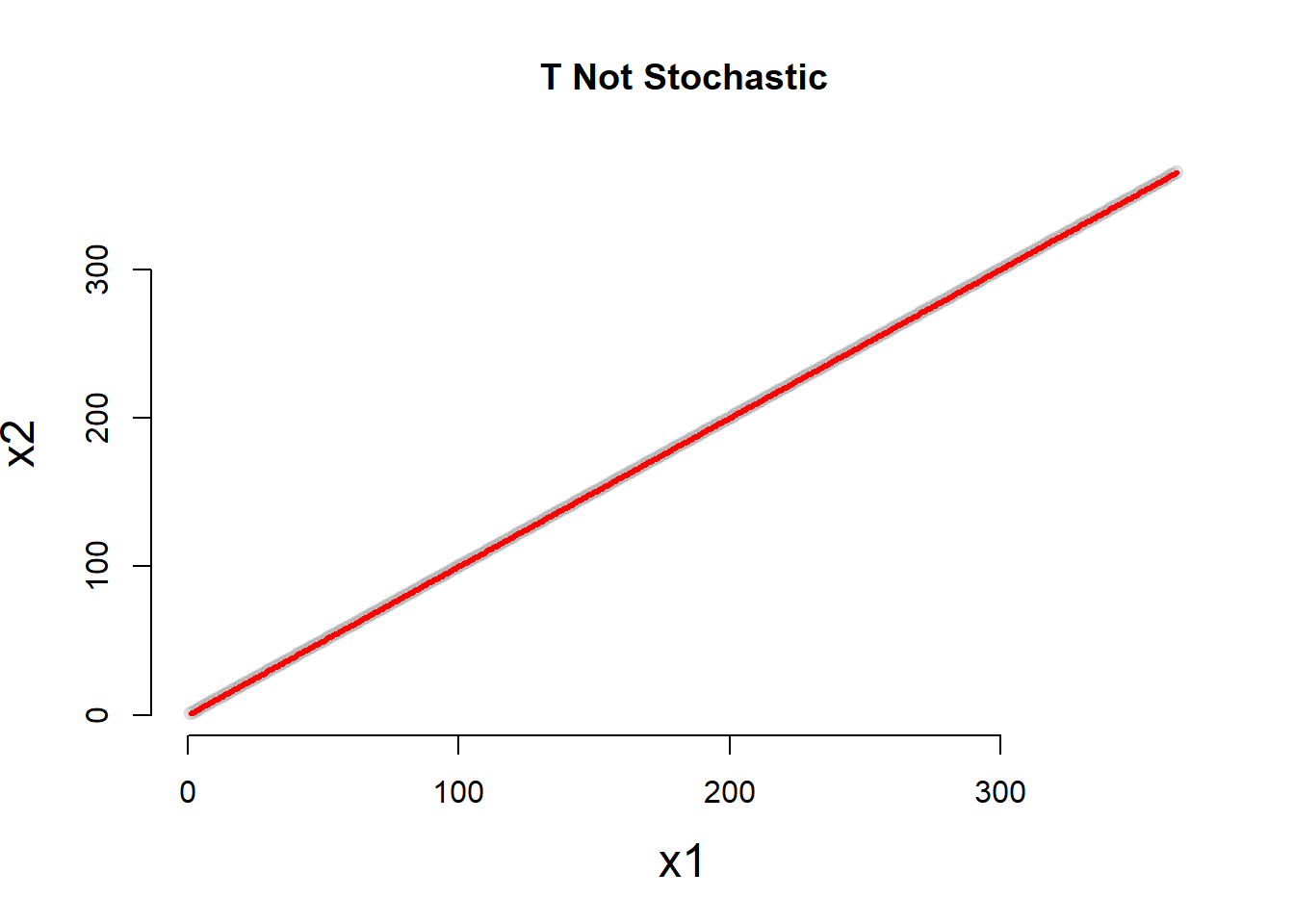table( dat1$D, dat2$D ) # not stochastic ## ## 0 1 ## 0 200 0 ## 1 0 165 par( mfrow=c(1,2) ) jplot( dat1$T, dat1$Y, main="Seed1" ) jplot( dat2$T, dat2\$Y, main="Seed2" )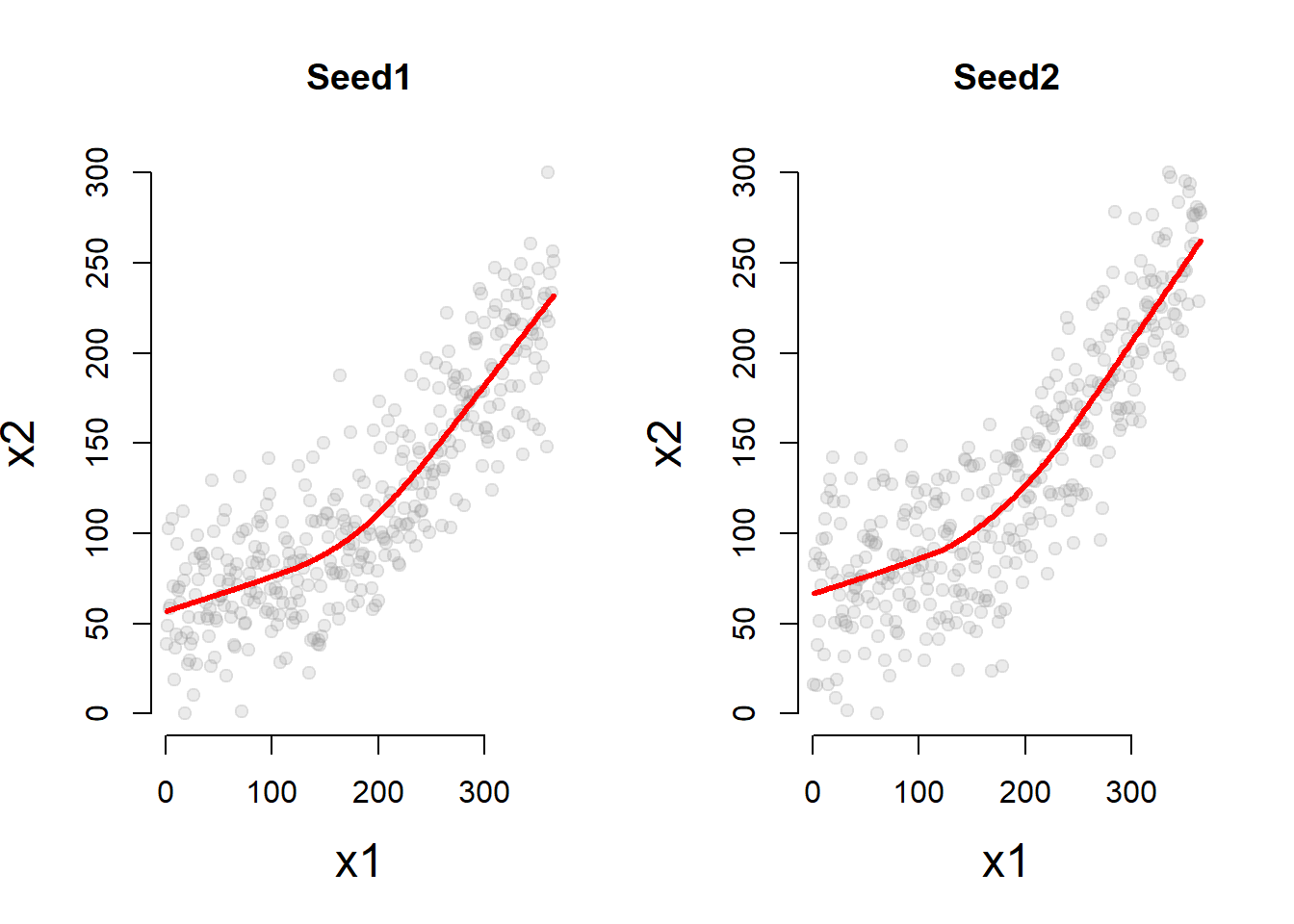reg1 = lm ( Y ~ T + D + P, data=dat1 )
reg2 = lm ( Y ~ T + D + P, data=dat2 )

stargazer( reg1, reg2,
type = "html",
dep.var.labels = ("Wellbeing Index"),
column.labels = c("Seed 1", "Seed 2"),
covariate.labels = c("Time", "Treatment", "Time Since Treatment"),
omit.stat = "all",
digits = 2 )
 Dependent variable: Wellbeing Index Seed 1 Seed 2 (1) (2) Time 0.19*** 0.18*** (0.04) (0.04) Treatment 13.09** 16.41** (6.12) (6.77) Time Since Treatment 0.54*** 0.68*** (0.06) (0.07) Constant 57.15*** 66.69*** (4.13) (4.56) Note: p<0.1; p<0.05; p<0.01